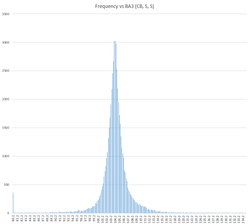top of page

# 2017 Cysteine Bond angle  Bulk Frequency PlotsBond 1: 110.2

Bond 2: 113.8

Bond 3: 104.2

# 2017 bulk data

These values are hand measurements of the disulfide bridges obtained from the R program. Each chi value has a corresponding frequency plot/histogram below that shows how many chi values have a specific bond angle in the data set. You can then observe trends in the chi values to determine the most common angles that can then be used to make the 3D model.

# 2016 Cysteine Bond angle Bulk Frequency Plots### The frequency plot derived from the R-program data for bond angles (N, CA, CB).### The frequency plot derived from the R-program data for bond angles (CA, CB, S).# 2016 Cysteine bulk data

This document has the measurements of the disulfide bridges obtained from the R program (approx. 15,000 proteins). Each chi value has a corresponding frequency plot/histogram below that shows how many angles have a specific bond angle in the data set. Here it is easier to see the more common bond angles because of the larger sample size.

Note: Some of the Chi2 bond angles did not properly run in the program and yielded "NaN" instead of bond angles in the data. In the above data set, those values were manually eliminated from the data set. Below is the unrefined data set. The cause of the issue is unknown.

bottom of page